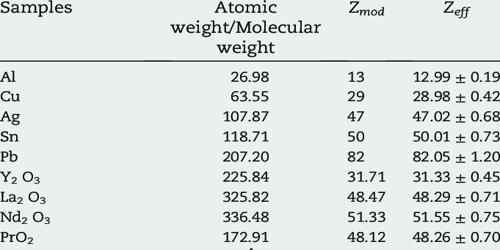Chemistry

# Effective Atomic NumberEffective atomic number (EAN), a number that represents the total number of electrons surrounding the nucleus of a metal atom in a metal complex. The effective atomic number has two different meanings: one that is the effective nuclear charge of an atom, and one that calculates the average atomic number for a compound or mixture of materials. Both are abbreviated Zeff. It is composed of the metal atom’s electrons and the bonding electrons from the surrounding electron-donating atoms and molecules.

For an atom

The sum of the number of electrons, donated by all ligands and those present on the central metal ion or atom in the complex is called an effective atomic number. The effective atomic number Zeff, (sometimes referred to as the effective nuclear charge) of an atom is the number of protons that an electron in the element effectively ‘sees’ due to screening by inner-shell electrons. Generally, EAN of central metal ion will be equal to the number of electrons in the nearest noble gas. It is a measure of the electrostatic interaction between the negatively charged electrons and positively charged protons in the atom. If the EAN of the central metal is equal to the number of electrons in the nearest noble gas then the complex possesses greater stability.

It is composed of the metal atom’s electrons and the bonding electrons from the surrounding electron-donating atoms and molecules. The binding energy of an electron, or the energy needed to remove the electron from the atom, is a function of the electrostatic interaction between the negatively charged electrons and the positively charged nucleus. In iron, atomic number 26, for instance, the nucleus contains 26 protons. Thus the effective atomic number of the cobalt atom in the complex [Co(NH3)6]3+ is 36, the sum of the number of electrons in the trivalent cobalt ion (24) and the number of bonding electrons from six surrounding ammonia molecules, each of which contributes an electron pair (2 × 6 =12).

Effective atomic numbers are useful not only in understanding why electrons further from the nucleus are so much more weakly bound than those closer to the nucleus but also because they can tell us when to use simplified methods of calculating other properties and interactions. It is the total number of electrons around the central metal ion present in a complex and calculated as the sum of the electrons on the metal ion and the number of electrons donated by the ligands. For instance, lithium, atomic number 3, has two electrons in the 1s shell and one in the 2s shell.

The effective atomic numbers (Zeff) depends on the range of atomic numbers of elements in the compound. Mathematically, the effective atomic number Zeff can be calculated using methods known as “self-consistent field” calculations, but in simplified situations is just taken as the atomic number minus the number of electrons between the nucleus and the electron being considered. Zeff value depends on the photon energy and buildup factor.